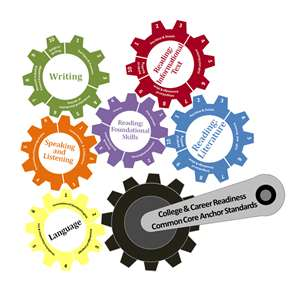Definitions of terms necessary for instruction:

Explain: Explain verbally or by showing understanding with manipulatives or with drawings.

Attribute: Characteristic, feature, element, or part of an area of math.

Interpret: Explain verbally or in writing.

Justify: Show process and/or prove correctness.

Understand:  Justify in a way appropriate to a student’s mathematical maturity why a math statement is true or where it comes from

 Sixth Grade District 15 Standards 2012/2013 Folder Links Page Links 6.G.1 I can find the area of triangles and quadrilaterals, and use those strategies to find the area of any polygon. •_Shapes • NavigatingThroughGeometry 1. Designing Bumper Cars 2. Changing Area, Changing Perimeter 3. Measuring Triangles 4. Measuring Parallelograms 5. Measuring Irregular Shapes 6.G.2 I can find the volume of a right rectangular prism with fractional edge lengths. •_Shapes • NavigatingThroughGeometry P 6.NS.2 I can fluently divide multi-digit numbers. •_Numbers 1. Multi-digit Divisions 6.NS.3 I can fluently solve multi-digit decimal problems using all four basic operations. •_Numbers 1. Decimals More or Less 2. Decimal Times 3. The Decimal Divide 4. Using Percents 5. More About Percents 6.G.4 I can represent a 3-D figure using a net and find the surface area. •5-23VolumeOfSolids • NavigatingThroughGeometry P 6.RP.1 I can describe and explain a ratio between two quantities. •_Ratios • MathCore/Fractions 1. Fundraising Fractions 2. Sharing And Comparing with Fractions 6.RP.2 I can determine and explain a unit rate in a given ratio. •Speed Math • MathCore/Fractions 1. Fundraising Fractions 2. Sharing And Comparing with Fractions 6.RP.3 I can use ratio and rate reasoning to solve real world and mathematical problems. •Speed Math • MathCore/Fractions 1. Estimating With Fractions 2. Adding and Subtracting Fractions 3. Multiplying With Fractions 4. Dividing With Fractions 6.NS.1 I can divide fractions with like and unlike denominators. •_Fractions • MathCore/Fractions 1. Estimating With Fractions 2. Adding and Subtracting Fractions 3. Multiplying With Fractions 4. Dividing With Fractions 6.NS.4a I can determine the GCF of two whole numbers from 1 to 100. •Factoring • EdHelper/Numbers P 6.NS.4b I can determine the LCM of two whole numbers from 1 to 12. •Factoring P 6.NS.4c I can use the Distributive Property to express the sum of two whole numbers from 1 to 100. •PropertiesIdentities P 6.NS.5 I can identify positive and negative value of quantities when given a real-world situation. •_Numbers P 6.NS.6 I can locate positive and negative rational numbers on a number line and use it to create a coordinate graph. •_Numbers 1. Fundraising Fractions 2. Sharing And Comparing with Fractions3. Moving between Fractions and Decimals 4. Working With Percents 1. Looking at Data 2. Using Graphs To Explore Data 3. What Do We Mean By Mean 6.NS.7 I can order and compare rational numbers and their absolute values when given a real-world situation. •_Numbers 1. Fundraising Fractions 2. Sharing And Comparing with Fractions 6.NS.8 I can apply real-world context to graph points in all four quadrants of the coordinate graph. •_Data 1. Looking at Data 2. Using Graphs To Explore Data 3. What Do We Mean By Mean 6.EE.1 I can write and solve problems using order of operations. •_Operations • OpsProps 1. Order of operations. (PEMDAS) 6.EE.2 I can translate and evaluate a relationship given in words into an algebraic expression. • Algebra • NavigatingThroughAlgebra 2. Variable Expressions 6.EE.3 I can apply the properties of operations to create equivalent expressions. • Algebra • NavigatingThroughAlgebra 3. Equivalent Expressions 6.EE.4 I can identify and prove when two expressions are equivalent. • Algebra • NavigatingThroughAlgebra 3. Equivalent Expressions 6.G.3 I can draw and find the side lengths of polygons in the coordinate graph when given coordinates for the vertices. •_Shapes 1. Bees and Polygons 2. Polygons and Angles 3. Polygon Properties and Tiling 4. Building Polygons 6.EE.5 I can explain that solving an equation or inequality leads to finding the value(s) of the variable that will make a true mathematical statement by using substitution. •Variables P 6.EE.6 I can use a variable to represent a mathematical or real-world situation. •Variables 2. Variable Expressions 6.EE.7 I can use inverse operations to solve one-step mathematical or real-world problems. •_Data 2. Variable Expressions 6.EE.8 I can write an inequality, explain the solution set, and graph on a number line. •_Data P 6.EE.9 I can create a table of two variables that represent the real-world situation in which one quantity will change in relation to the other. •_Data P 6.SP.1 I can recognize and write statistical questions that will include variability. •_Data 1. Looking at Data 2. Using Graphs To Explore Data 3. What Do We Mean By Mean 6.SP.2 I can describe a set of data by its center, spread, and overall shape. •_Data 1. Looking at Data 2. Using Graphs To Explore Data 3. What Do We Mean By Mean 6.SP.3 I can represent and explain the difference between measures of center and measures of variability. •_Data 1. Looking at Data 2. Using Graphs To Explore Data 3. What Do We Mean By Mean 6.SP.4 I can display data using a number line, dot plot, histogram, and box plots. •_Data 1. Looking at Data 2. Using Graphs To Explore Data 3. What Do We Mean By Mean 6.SP.5 I can summarize numerical data sets in relation to their context. •_Data 1. Looking at Data 2. Using Graphs To Explore Data 3. What Do We Mean By Mean

Major clusters require greater emphasis based on depth of ideas, time they take to master, and/or importance to future mathematics.

Support clusters.